Measure Mass & Volume (Metric) Math Videos for Kids - Grades 3-5
1%
It was processed successfully!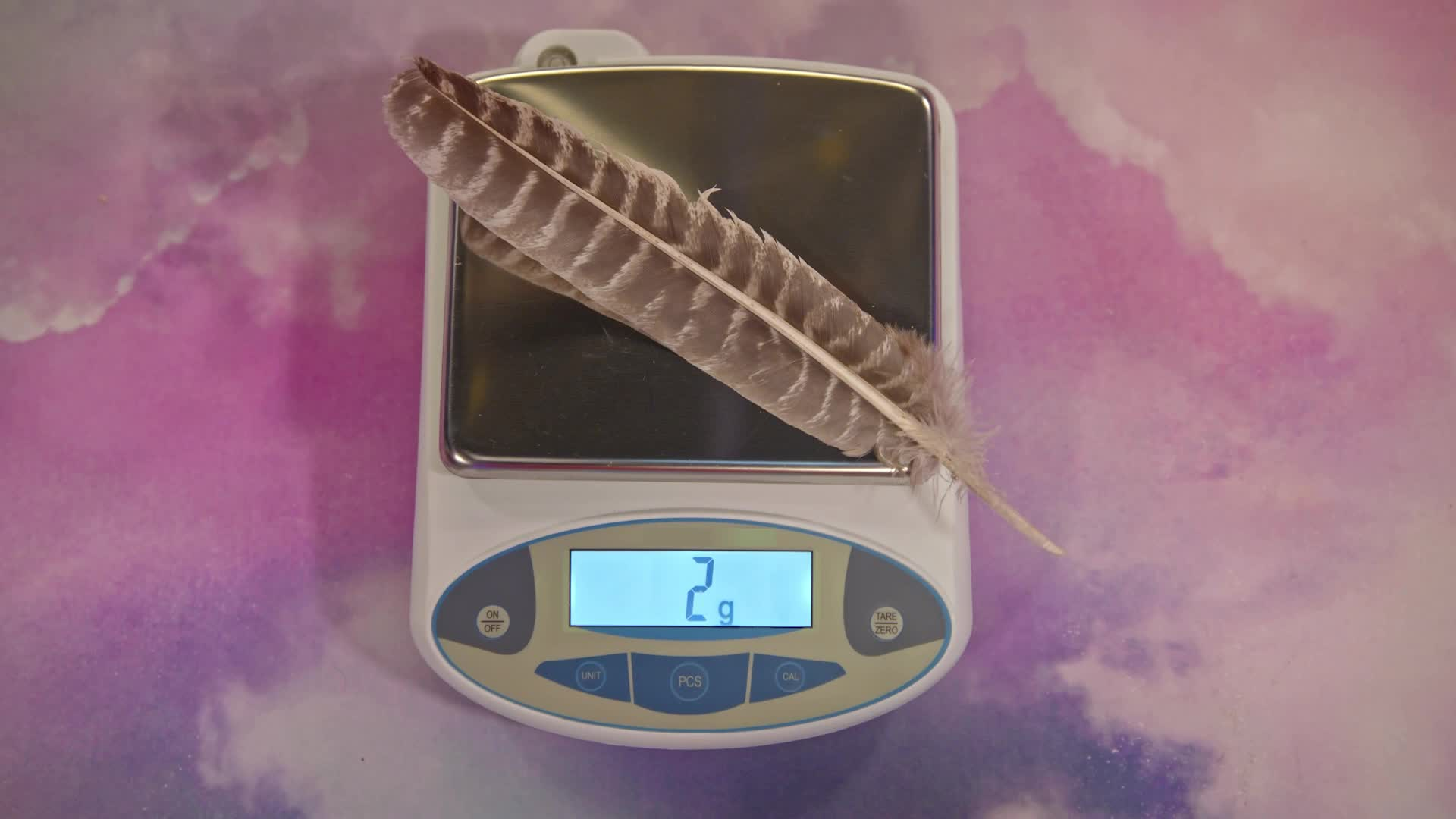WHAT IS MEASURING MASS & VOLUME?

Grams (g) and kilograms (kg) are used to measure mass. Liters (L) and milliliters (mL) are used to measure volume.

To better understand measuring mass & volume…

WHAT IS MEASURING MASS & VOLUME?. Grams (g) and kilograms (kg) are used to measure mass. Liters (L) and milliliters (mL) are used to measure volume. To better understand measuring mass & volume…

## LET’S BREAK IT DOWN!

### Cooking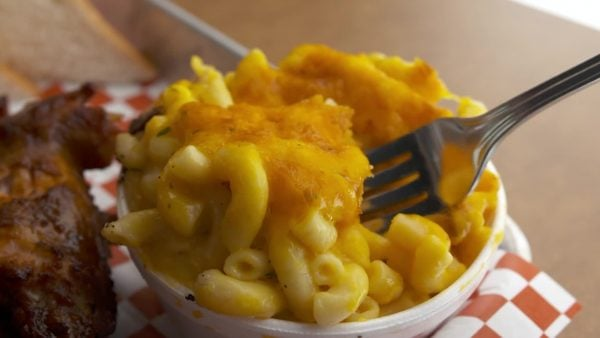Let’s say you want to make macaroni and cheese. You need 500 grams of shredded cheese and 280 grams of macaroni. What is the total mass of these ingredients? You can add measurements just like regular numbers to find out. 500 grams + 280 grams = 780 grams. The total mass is 780 grams. Try this one yourself. Suppose you want to make a batch of macaroni that is a little bit bigger. It will have 750 grams of shredded cheese and 420 grams of macaroni. What is the total mass of these ingredients?

Cooking Let’s say you want to make macaroni and cheese. You need 500 grams of shredded cheese and 280 grams of macaroni. What is the total mass of these ingredients? You can add measurements just like regular numbers to find out. 500 grams + 280 grams = 780 grams. The total mass is 780 grams. Try this one yourself. Suppose you want to make a batch of macaroni that is a little bit bigger. It will have 750 grams of shredded cheese and 420 grams of macaroni. What is the total mass of these ingredients?

### Sports Drink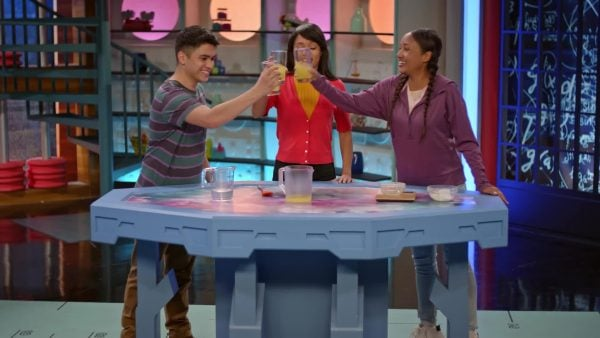Let’s say you want to make a sports drink. You have 1,000 milliliters of water but you only need 750 milliliters of water to make the sports drink. How much water will you have left after you make the drink? You can subtract measurements just like regular numbers to find out. 1,000 mL – 750 mL = 250 mL. Try this one yourself. You have 2,000 milliliters of water but you only need 1,500 milliliters of water to make a sports drink. How much water will you have left after you make the sports drink?

Sports Drink Let’s say you want to make a sports drink. You have 1,000 milliliters of water but you only need 750 milliliters of water to make the sports drink. How much water will you have left after you make the drink? You can subtract measurements just like regular numbers to find out. 1,000 mL – 750 mL = 250 mL. Try this one yourself. You have 2,000 milliliters of water but you only need 1,500 milliliters of water to make a sports drink. How much water will you have left after you make the sports drink?

### Planting Seeds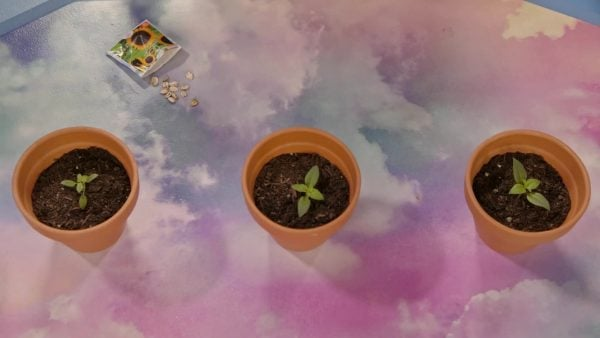Let’s say you have three pots and each pot holds 210 grams of soil. How much soil do you need to fill three pots? You can find the total by multiplying 3 by 210 grams. 210 grams × 3 = 630 grams. You need 630 grams of soil to fill three pots. Try this one for yourself. You have five pots and each pot holds 300 grams of soil. How much soil do you need to fill five pots?

Planting Seeds Let’s say you have three pots and each pot holds 210 grams of soil. How much soil do you need to fill three pots? You can find the total by multiplying 3 by 210 grams. 210 grams × 3 = 630 grams. You need 630 grams of soil to fill three pots. Try this one for yourself. You have five pots and each pot holds 300 grams of soil. How much soil do you need to fill five pots?

### Candy in a Piñata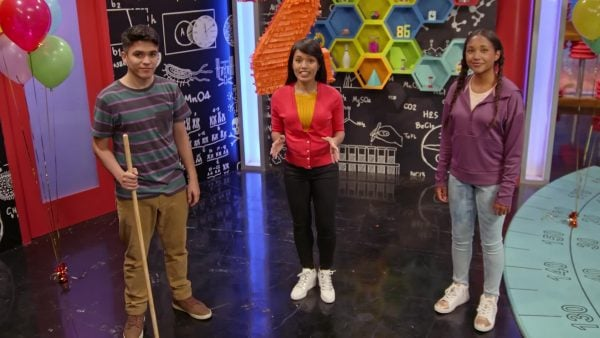Let’s say you have a piñata with 600 grams of candy inside. You want to divide the candy evenly between you and your two friends. How many grams of candy do each of you get? You can divide the candy into three equal groups to find the answer. 600 ÷ 3 = 200. You each get 200 grams of candy. Try this one for yourself. You have a piñata with 800 grams of candy. You want to divide the candy evenly between you and one of your friends. How many grams of candy do each of you get?

Candy in a Piñata Let’s say you have a piñata with 600 grams of candy inside. You want to divide the candy evenly between you and your two friends. How many grams of candy do each of you get? You can divide the candy into three equal groups to find the answer. 600 ÷ 3 = 200. You each get 200 grams of candy. Try this one for yourself. You have a piñata with 800 grams of candy. You want to divide the candy evenly between you and one of your friends. How many grams of candy do each of you get?

## MEASURING MASS & VOLUME VOCABULARY

Milliliter
A unit used to measure liquid volume.
Gram
A unit used to measure mass.
Mass
A measure of how much matter an object contains.
Volume
A measure of how much space an object or liquid takes up.
Beaker
A cylindrical container used in science.
Kilogram
A larger unit used to measure mass. Every 1,000 grams can be grouped into 1 kilogram.
A larger unit to measure volume. Every 1,000 milliliters can be grouped into 1 liter.
A system of measurement that uses milliliter, liter, gram, and kilogram as measuring units along with other measuring units.

## MEASURING MASS & VOLUME DISCUSSION QUESTIONS

### What is mass and what are some of the units we use in the metric system to measure mass?

Mass is a measure of how much matter an object contains. We use grams and kilograms to measure mass.

### What is liquid volume and what are some of the units we use in the metric system to measure liquid volume?

Liquid volume is a way to measure the amount of liquid by giving a measurement of how much space it occupies. We use liters and milliliters to measure liquid volume.

### What unit would you use to measure the mass of an elephant, grams or kilograms? Why?

An elephant is large and contains a lot of matter. If I used grams, the number of grams would be gigantic. If I use kilograms, I will get a number of kilograms that is easier to work with.

### What unit would you use to find the liquid volume of the water in an eyedropper, liters or milliliters? Why?

The amount of water in an eyedropper is very small so you would want to use a milliliter which is a small unit of liquid volume. A liter is a unit that would be too big.

### A scientist needs to put 400 grams of salt into a container. She has already put 175 grams of salt into the container so far. How many more grams of salt does she need to put into the container? Explain how you solved the problem.

The scientist needs to subtract the amount of salt she has already put into the container from the total amount of salt she needs to put into the container. 400 – 175 = 225. She needs to put 225 more grams of salt into the container.
X

## Success

We’ve sent you an email with instructions how to reset your password.
Ok

## Choose Your Free Trial Period### 7 DaysContinue to Lessons### 90 DaysGet 90 days free by inviting other teachers to try it too.

Share with Teachers

## Get 90 Days Free

### By inviting 4 other teachers to try it too.

4 required

Skip, I will use a 7 days free trial

## Thank You!

Enjoy your free 90 days trial